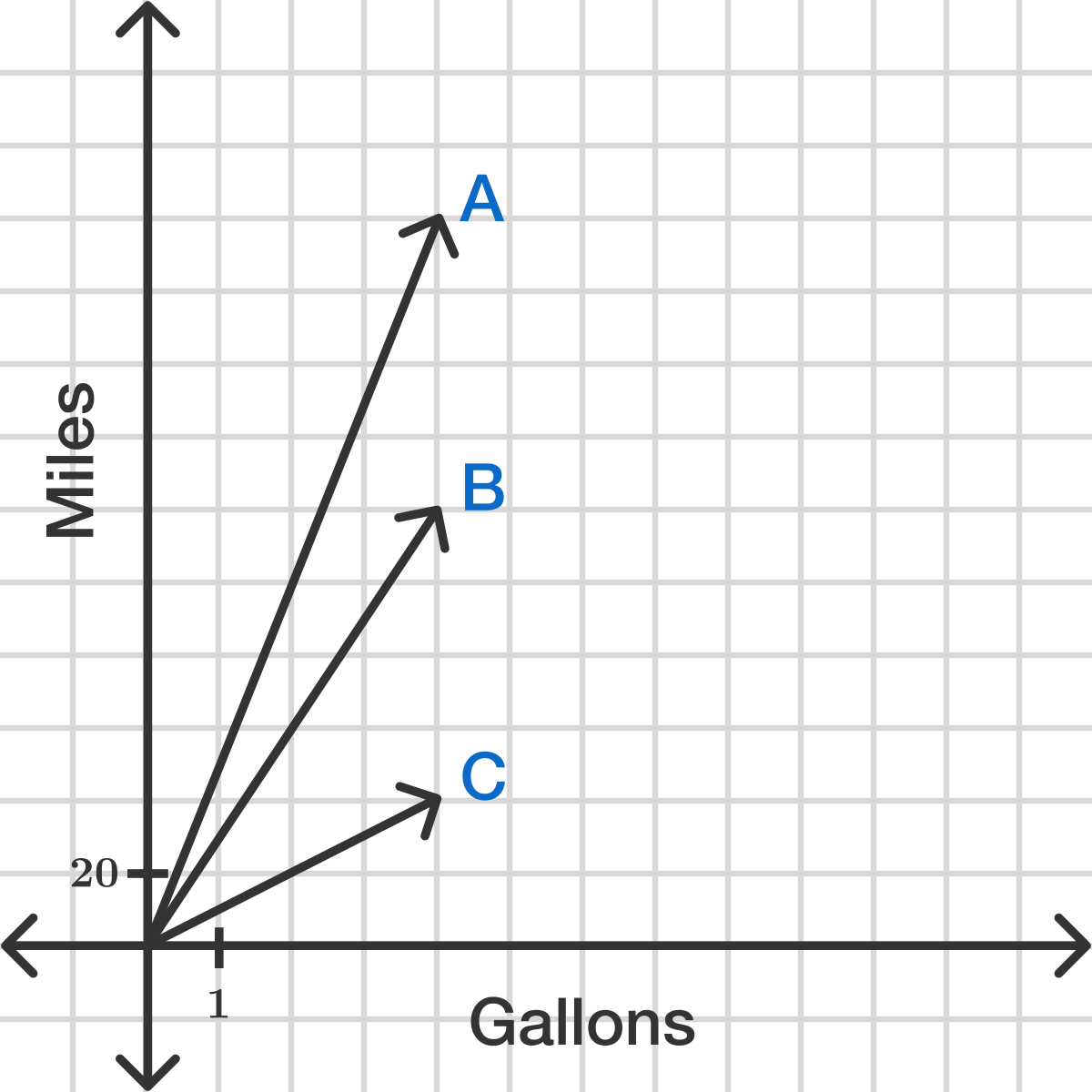# Confuse with the solution shown in the rates of change

Hi, I just to know why the solution of this last problem in rates of change for geometry is given like this:

Let each driver drives m miles per week. The fuel used by car A is m/10 The fuel used by car B is m/30 The fuel used by car C is m/50.

Stan saves m/10 - m/30 = m/15 gallons per week.

Ximena saves m/30 - m/50 = m/75 gallons per week.This is the question being given: "Stan and Ximena both want more fuel efficient vehicles. Stan is going to switch from driving car C to car B and Ximena is going to switch from driving car B to car A. Is the following statement true or false?

Stan and Ximena will save the same amount of fuel."

I am really confuse as to why car A is m/10, car B is m/30, and car C is m/50. Even it says each represent m miles per week. Thanks for the help.3 years, 2 months ago

This discussion board is a place to discuss our Daily Challenges and the math and science related to those challenges. Explanations are more than just a solution — they should explain the steps and thinking strategies that you used to obtain the solution. Comments should further the discussion of math and science.

When posting on Brilliant:

• Use the emojis to react to an explanation, whether you're congratulating a job well done , or just really confused .
• Ask specific questions about the challenge or the steps in somebody's explanation. Well-posed questions can add a lot to the discussion, but posting "I don't understand!" doesn't help anyone.
• Try to contribute something new to the discussion, whether it is an extension, generalization or other idea related to the challenge.

MarkdownAppears as
*italics* or _italics_ italics
**bold** or __bold__ bold
- bulleted- list
• bulleted
• list
1. numbered2. list
1. numbered
2. list
Note: you must add a full line of space before and after lists for them to show up correctly
paragraph 1paragraph 2

paragraph 1

paragraph 2

[example link](https://brilliant.org)example link
> This is a quote
This is a quote
    # I indented these lines
# 4 spaces, and now they show
# up as a code block.

print "hello world"
# I indented these lines
# 4 spaces, and now they show
# up as a code block.

print "hello world"
MathAppears as
Remember to wrap math in $$ ... $$ or $ ... $ to ensure proper formatting.
2 \times 3 $2 \times 3$
2^{34} $2^{34}$
a_{i-1} $a_{i-1}$
\frac{2}{3} $\frac{2}{3}$
\sqrt{2} $\sqrt{2}$
\sum_{i=1}^3 $\sum_{i=1}^3$
\sin \theta $\sin \theta$
\boxed{123} $\boxed{123}$

Sort by:

I assume you are referring to this problem.

$m/10$ represents the amount of fuel used by car A is per mileage....

- 3 years, 2 months ago

Yes.

- 3 years, 2 months ago